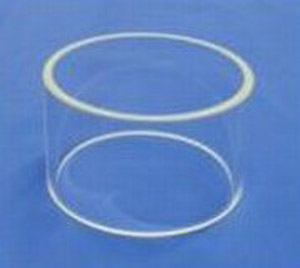A# cylinderA cylinder is a three-dimensional surface described by the Cartesian equation (x/a)2 + (y/b)2 = 1. If a = b then the surface is a circular cylinder, otherwise it is an elliptic cylinder.

The cylinder is a degenerate quadric because at least one of the coordinates (in this case z) doesn't appear in the equation, though by some definitions the cylinder isn't considered to be a quadric at all. In common usage, a cylinder is taken to mean a finite section of a right circular cylinder with its ends closed to form two circular surfaces.

If the cylinder has a radius r and a length h, then its volume is V = πr 2h and its surface area is A = 2πr 2 + 2πrh. For a given volume, the cylinder with the smallest surface area has h = 2r. For a given surface area, the cylinder with the largest volume has h = 2r. More unusual types of cylinder include the imaginary elliptic cylinder: (x/a)2 + (y/b)2 = -1, the hyperbolic cylinder: (x/a)2 - (y/b)2 = 1, and the parabolic cylinder: x2 + 2y = 0.